ChaptersThis text is meant to accompany class discussions. It is not everything there is to know about uniform circular motion. It is meant as a  prep for class. More detailed notes and examples are given in the class notes, presentations, and demonstrations (click here.)

Table of Contents
 Page Description 0 Objectives (scroll down) 1 Horizontal Motion 2 Vertical Motion 3 Motion in a Plane (Position) 4 Motion in a Plane (Organization and Direction) 5 Projectile Motion 6 Projectile Motion Givens with Trigonometry 7 Steps to Solving Projectile Motion Problems 8 Implied Givens and Other Short Cuts 9 Calculating Impact Velocity's Magnitude and Direction

Objectives
Students should be able to:
1 Draw and label a picture of the problem showing numbers and units
2 Draw a triangle showing the initial velocity, its angle, and its horizontal and vertical components.
3 Draw this triangle with vectors.
4 List givens with units in a table.
5 Describe what is being calculated.
6 Show the equation with only variables.
7 Isolate the unknown showing only the letter variables.

8

Show equations with the numbers AND units.

9

Show the answer with the correct sig. figs. and units
10 Calculate and the following given the initial velocity, (magnitude and direction,) and one other "given."

 a Height to apogee b Time to apogee c Time in the air d Range e Landing height f Impact velocity (magnitude and direction,) with a vector triangle of the impact velocity's components. g Given the range you can calculate the height. h Given the height, you can calculate the range. i Directions and signs of the variables.
11 You will do this involving problems that set up like any of the following for problems where the initial velocity is up or down at an angle

 a Lands at the same height as the start height. b Lands higher than the start height. c Lands lower than the start height. d Rolls horizontally off a surface to a landing below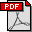Handouts/Worksheets are in the workbook under the projectile motion section.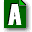ANSWERS to workbook problems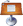Lecture Notes L Projectile Motion Investigator Activity L Projectile Motion " Which Lands First?" Digital Activity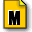Mastery assignment L Projectile Lab - ActivityReview QuizTest Review Questions and Answers in One Document (293 kb) Help Videos on YouTube ...by me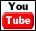Identifying the givens and breaking up the initial velocity into vertical and horizontal pieces.Doing calculations with apogeeDetermining the signs of the variablesHow to organize your givens in a tableOne method for find the time in flightCalculating an impact velocities magnitude and direction (honors only)Lab - activity set up videoLab - collecting data using a photogate, LabPro2, and the TI-84 Calculator

by Tony Wayne ...(If you are a teacher, please feel free to use these resources in your teaching.)